# Automata - Finite Automata

A finite automaton is an automaton that has a set of states and its control moves from state to state in response to external inputs.

It has a start and an end state and there are only a finite number of states

## Definition

A formal de definition of a finite automaton is whenever:

• an input X is received by an automaton,
• the automaton must follow an arc labeled X from the state it is in to some new state (for all states)

## Example

### Lexer

An automaton implementing a lexer modeling the recognition of the keyword then

where:

• the state (circle) represents a different position in the word then that has been reached so far that ranges from:
• the empty string (ie nothing)
• to the complete word

## Implementation

The advantage of having only a finite number of states is that we can implement the system with a fixed set of resources

Example:

• Hardware: we could implement it in hardware as a circuit
• Software: to make the decision
• looking at the state
• or using the position in the code itself

### Model

example: A on/off button

## Documentation / Reference

Recommended Pages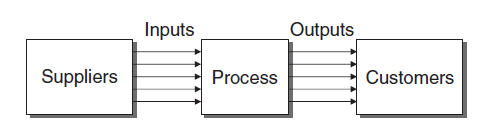A business process is anatural process (ie versus machine process) where activities are performed in a organisation. Example business process : raw materials purchasing orders shipments invoicing...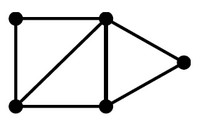(Network|Graph) - Finite

See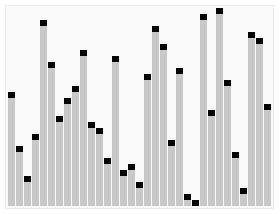Algorithm

An is a (procedure|method) for solving a problem. If there exists an algorithm, the function that performs it is called computable. Study of algorithms dates at least to Euclid and were formalized by...Automata - Deterministic finite-state automata (DFA)

A Deterministic finite-state automata (DFA) is a finite automaton that cannot be in more than one state at any one time. The term deterministic refers to the fact that on each input there is one and only...Automata - Nondeterministic automata (NFA)

Nondeterministic finite automata (NFA) is a finite automata that can be in several states at once (several variable) The inverse of Nondeterministic automata (NFA) is deterministic finite-state automata...Automaton (State Machine)

Automaton (State Machine) Automata theory is the study of abstract computing devices or machines. The word automata (the plural of automaton) comes from the Greek word αὐτόματα, which means...Data Processing - (Pipeline | Compose | Chain)

A pipeline is a finite automata where: the data transition from one state to another via a series of transformations (work) A pipeline creates a composition relationship. A pipeline is also...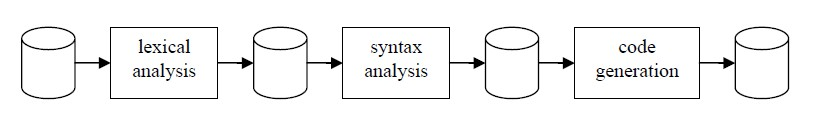Grammar - Hierarchy of grammar (Chomsky)

In the formal languages (of computer science and linguistics), the Chomsky hierarchy is a hierarchy of formal grammars described by Noam Chomsky in 1956. The hierarchy describes the relations between:...Lexical Analysis - (Token|Lexical unit|Lexeme|Symbol|Word)

A token is symbols of the vocabulary of the language. Each token is a single atomic unit of the language. The token syntax is typically a regular language, so a finite state automaton constructed from...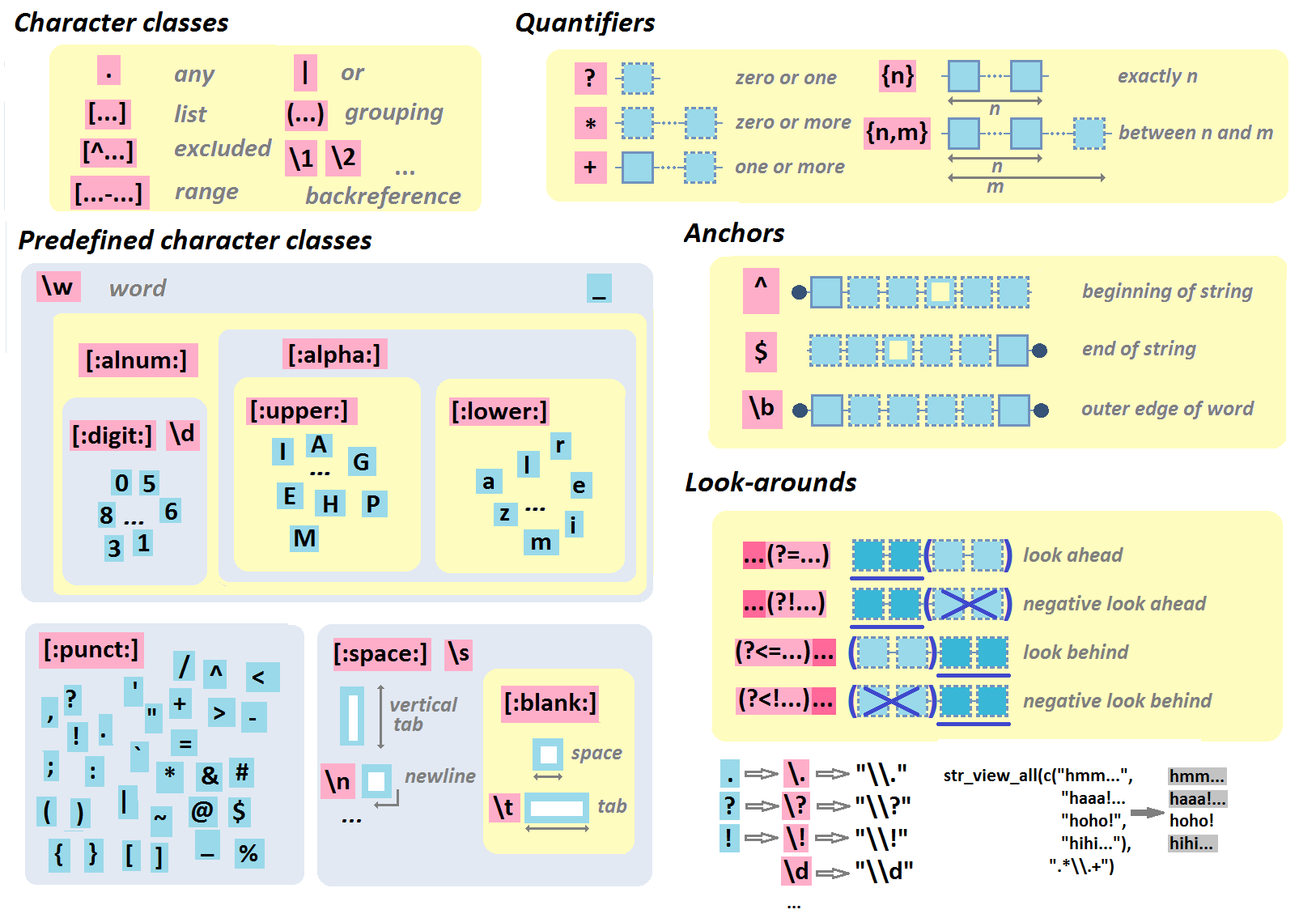Multilingual Regular Expression Syntax (Pattern)

Regular expression are Expression that defines a pattern in text. This is therefore a language that permits to define structure of a text. They are a mathematically-defined concept, invented by Stephen...